# Python Issue: Using Arithmetic Operation [Solved]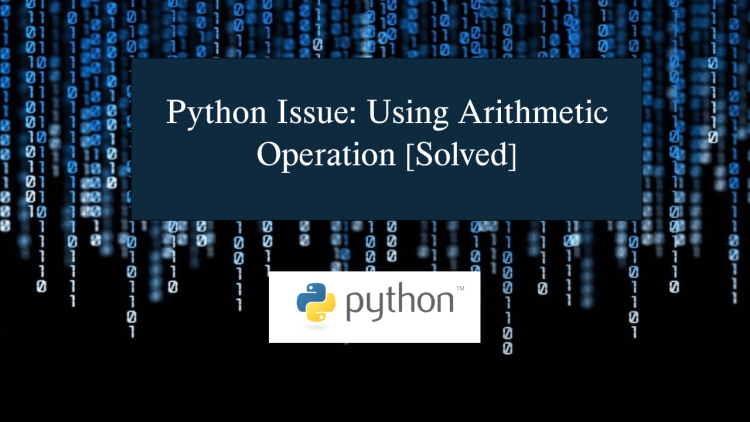In this article, we will delve into the fundamental use of arithmetic functions in Python 3. The main focus is on addressing the issue of Python Issue: Using Arithmetic Operation. We will provide effective solutions to resolve this problem and explore Python programming in-depth to prevent encountering similar issues in the future. If you are looking for a specific solution to any errors or are unable to make your code work, this article might help you solve your problem.

### What is Arithmetic Operation?

Arithmetic operations is a branch of mathematics that involves the study of numbers and their operations, which are fundamental in all other branches of mathematics. It primarily encompasses addition, subtraction, multiplication, and division.

In this article, we will discuss the fundamental use of arithmetic operation in python programming. I will provide a sample code to demonstrate how to use the arithmetic operation in a python script.

In Python programming, the addition and subtraction operators function very similarly to their mathematical counterparts. Python can also serve as a calculator, allowing for straightforward arithmetic operations.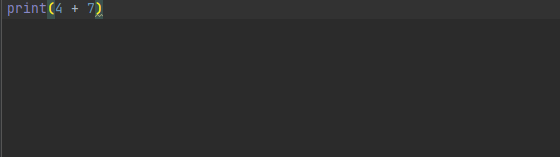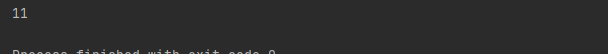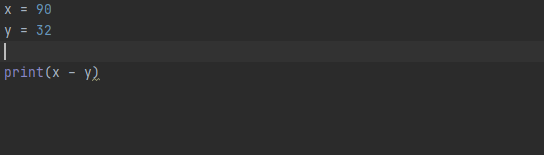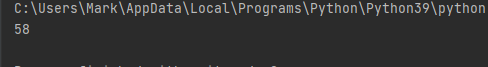## Multiplication and Division

Similar to addition and subtraction, multiplication and division in Python closely resemble their mathematical counterparts. In Python, the asterisk symbol * is used for multiplication, and the forward slash / is used for division.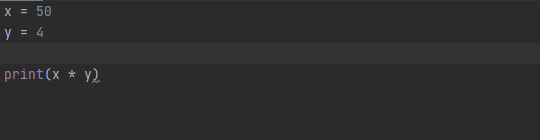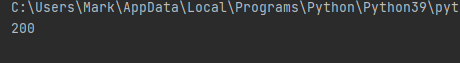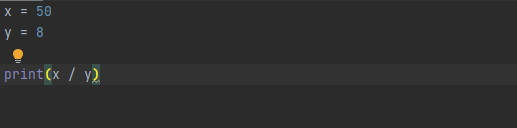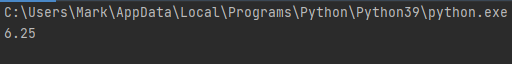## Conclusion

The Python Issue: Using Arithmetic Operation [Solved] is a common problem that many developers encounter when working with arithmetic operations. These errors often result from the misuse or incorrect placement of variables.